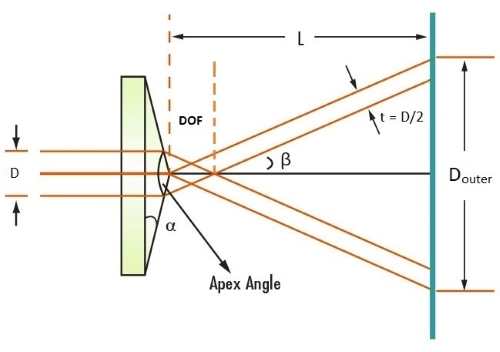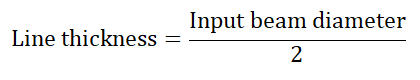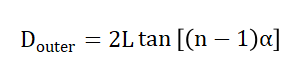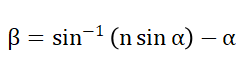# Axicon Calculator

This is an online calculator to find the half fan angle, depth of focus, outer diameter, and line thickness of the axicon. Just enter the diameter of the beam that enters the lens, length from axicon to image formed, the refractive index of the axicon, and the axicon angle value.

• mm
• mm
• Degrees

### Result

• Depth of Focus
mm
• Outer Diameter
mm
• Line Thickness
mm
• Half Fan Angle
Degrees
• Apex Angle
Degrees
##### Image for Axicon CalculatorAxicon is an optical device identical to a lens. One side of it is conical and the other side is flat, and thus it is also known as a Conical lens or Conical prism. Axicons do not focus the beam of light to a point; instead, it uses interference to form a focal plane along the optical axis.When a parallel beam of light passes through an axicon, due to refraction, the output rays formed are conical and they interfere with each other. Within this beam overlap region, the axicons have properties of a Bessel beam, which are beams that comprise rings that are non-diffracting. So an axicon can be used to convert a Gaussian laser beam into a non-diffracting Bessel beam.

Ideally, a Bessel beam is a beam having an infinite number of rings covering infinite distances which are non-diffracting. This requires infinite energy which physically does not exist. So, Bessel beams are non-diffracting beams defined over an axial distance. They are known as ‘self-healing’ because if an object occurs at the center of the beam, it will not block the rays as they will interfere with each other to reform the beam. This beam is mathematically defined by the Bessel function.

The depth up to which the Bessel beam exists is known as the Depth Of Focus (DOF). It is given by,Where 'n' is the refractive index and 'α' is the axicon angle which is the inclination angle of the surface.

The interference of the conical rays results in a ring of light beyond the depth of focus. The thickness of this ring remains constant and is equivalent to the radius of the beam entering the axicon, i.e.The Outer Diameter of the ring increases with increasing length from lens output to the image. It is given by,Where 'L' is the length from the axicon to the plane.

The Half Fan Angle (β) after the beam passes through the axicon is given by,Apex angle is the angle made by the conical side of an axicon and is given by,